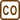## Thermal Insulation of a House

### Task number: 1278

Owners of an older block of flats with perimeter walls 20 cm thick have decided to save money on heating by insulating the house from the outside using a polystyrene insulation layer with a thickness of 10 cm. Consider the model example of a winter day with the outdoor temperature of −10 °C and the room temperature of 20 °C. Determine the temperature between the wall and polystyrene. What is the reduction factor of heat losses?

• #### Hint

To solve this task we need to use the thermal conduction equation, which indicates that the heat passing through the material is proportional to the temperature difference between both sides of the material.

Given that both materials - concrete and polystyrene - are in succession, the heat in steady state passing through the concrete must subsequently pass through the polystyrene "just as quickly". Otherwise, there would be changes of temperature between the two materials. We determine the temperature between the two materials from the fact, that the heat flow through the concrete must equal the heat flow through polystyrene.

• #### Numerical values

 d1 = 0.2 m tthe thickness of the wall d2 = 0.1 m the thickness of polystyrene insulation layer t1 = 20 °C the room temperature t2 = −10 °C the outdoor temperature t = ? the temperature between the wall and polystyrene Q1 /Q1= ? pthe ratio of the heat lost through the uninsulated and insulated wall, respectively From The Handbook of Chemistry and Physics: λ1 = 1.3 Wm−1K−1 the thermal conductivity coefficient of the wall (panel = concrete) λ2 = 0.1 Wm−1K−1 the thermal conductivity coefficient of polystyrene
• #### Analysis

At steady state, the heat which passed through the panel wall must equal the heat which passed through the polystyrene insulation. Since the amount of heat that passes through the material is proportional to the temperature differences between its both sides (assuming that these temperatures are constant), we can determine the temperature between the wall and the polystyrene using the equality of the passed heats.

The heat that passes through an insulated wall is equal to the heat that only passes through the wall at given difference between the room temperature and the temperature at the wall-insulation contact. It is also equal to the heat transferred through the polystyrene insulation at given difference between the outside temperature and the temperature at the wall-insulation contact.

• #### Solution

The thermal conduction equation is:

$Q=\lambda\frac{S\Delta t}{d}\tau,$

where Q is the heat transferred per time τ, λ is the thermal conductivity coefficient (characterizing the material), S is the surface area, d is the thickness of the material through which the heat passes and Δt is the temperature difference between both sides of the material, which remains constant.

To determine the temperature between the wall and the polystyrene insulation we use the fact that at steady state the heat transferred though the wall equals the heat transferred through the polystyrene insulation (otherwise the temperature between the wall and polystyrene would change):

$\lambda_1\frac{S\left(t_1-t\right)}{d_1}\tau=\lambda_2\frac{S\left(t-t_2\right)}{d_2}\tau$

We can thereof evaluate the unknown temperature t:

$d_2\lambda_1\left(t_1-t\right)=d_1\lambda_2\left(t-t_2\right)$ $d_2\lambda_1t+d_1\lambda_2t=d_2\lambda_1t_1+d_1\lambda_2t_2$ $t=\frac{d_2\lambda_1t_1+d_1\lambda_2t_2}{d_2\lambda_1+d_1\lambda_2}=\frac{0.1\cdot{1.3}\cdot{20}+0.2\cdot{0.1}\cdot(-10)}{0.1\cdot{1.3}+0.2\cdot{0.1}}\,\mathrm{^\circ C}=16\,\mathrm{^\circ C}$

To calculate the reduction factor of heat losses, we need to evaluate the heat, which is transferred through both uninsulated and insulated wall within the given time. An uninsulated wall:

$Q_1=\lambda_1\frac{S\left(t_1-t_2\right)}{d_1}\tau$

The heat transferred through an insulated wall equals the heat that passes through just the wall at smaller temperature difference:

$Q_2=\lambda_1\frac{S\left(t_1-t\right)}{d_1}\tau$

For t we now substitute the expression we have evaluated earlier:

$Q_2=\lambda_1\frac{S\left(t_1-\frac{d_2\lambda_1t_1+d_1\lambda_2t_2}{d_2\lambda_1+d_1\lambda_2}\right)}{d_1}\tau$ $Q_2=\lambda_1\frac{S\tau}{d_1}\frac{d_2\lambda_1t_1+d_1\lambda_2t_1-d_2\lambda_1t_1-d_1\lambda_2t_2}{d_2\lambda_1+d_1\lambda_2}$ $Q_2=\lambda_1\frac{S\tau}{d_1}\frac{d_1\lambda_2t_1-d_1\lambda_2t_2}{d_2\lambda_1+d_1\lambda_2}=\frac{\lambda_1\lambda_2\left(t_1-t_2\right)}{d_2\lambda_1+d_1\lambda_2}S\tau$

Note: We can see that both thermal conductivity coefficients λ1 and λ2 are in an equivalent position, so that we would get to the same expression if we evaluated the amount of heat transferred through the polystyrene (we derived the temperature t from the equality of both heats, therefore both heats must equal). This corresponds to the heat transferred through an insulated wall (wall + polystyrene).

Also, the resulting relationship gives us guidance on how to determine the "average thermal conductivity" for a composite material. If we would like to find such λ that could be used for a wall with insulation as a whole (total thickness d1 +  d2), then by comparing the obtained expression and the general thermal conduction equation we get: $\lambda\frac{S\left(t_1-t_2\right)}{d_1+d_2}\tau=\frac{\lambda_1\lambda_2\left(t_1-t_2\right)}{d_2\lambda_1+d_1\lambda_2}S\tau$ $\lambda=\frac{\lambda_1\lambda_2\left(d_1-d_2\right)}{d_2\lambda_1+d_1\lambda_2}$

Finally, we determine the ratio of both heats:

$\frac{Q_2}{Q_1}=\frac{\frac{\lambda_1\lambda_2\left(t_1-t_2\right)}{d_2\lambda_1+d_1\lambda_2}S\tau}{\lambda_1\frac{S\left(t_1-t_2\right)}{d_1}\tau}=\frac{0.2\cdot{0.1}}{0.1\cdot{1.3}+0.2\cdot{0.1}}=0.13=13\%\,$
• #### Answer

The temperature between the wall and the polystyrene is 16 °C and the heat loss decreases to 13 % of the original value after insulating the wall.